Courses

# Competition Level Test: Solution Of Triangles- 3

## 27 Questions MCQ Test Mathematics For JEE | Competition Level Test: Solution Of Triangles- 3

Description
This mock test of Competition Level Test: Solution Of Triangles- 3 for JEE helps you for every JEE entrance exam. This contains 27 Multiple Choice Questions for JEE Competition Level Test: Solution Of Triangles- 3 (mcq) to study with solutions a complete question bank. The solved questions answers in this Competition Level Test: Solution Of Triangles- 3 quiz give you a good mix of easy questions and tough questions. JEE students definitely take this Competition Level Test: Solution Of Triangles- 3 exercise for a better result in the exam. You can find other Competition Level Test: Solution Of Triangles- 3 extra questions, long questions & short questions for JEE on EduRev as well by searching above.
QUESTION: 1

### AA1, BB1 and CC1 are the medians of triangle ABC whose centroid is G. If points A, C1, G and B1 are concyclic, then

Solution:

AA1, BB1 and CC1 are the medians of triangle ABC whose centroid is G.
Since A, C1, G and B1 are concyclic, then
BG⋅BB1 = BC1⋅BA
2/3 ( BB1)2 = (c/2).c
(2/3).(1/4).(2a2 + 2c2 - b2) = c2/2
2a2 + 2c2 - b2 = 3c2
b2 + c2 = 2a2

QUESTION: 2

Solution:
QUESTION: 3

### In a ΔABC, a = 1 and the perimeter is six times the A.M. of the sines of the angles. Then measure of ∠A is

Solution:
QUESTION: 4

If the median AD of a triangle ABC divides the angle ∠BAC in the ratio 1 : 2, then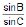is equal to

Solution:
QUESTION: 5

In a triangle ABC, let ∠C = π/2, if r is the inradius and R is the circumradius of the triangle ABC, then 2(r + R) equals

Solution:
QUESTION: 6

If in a ∠ABC, the altitudes from the vertices A, B, C on opposite sides are in HP, then sinA, sinB, sinC are in

Solution:
QUESTION: 7

The sides of a triangle are sina, cosa, and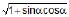for some 0 < a < π/2. Then the greatest angle of the triangle is

Solution:
QUESTION: 8

The sum of the radii of inscribed and circumscribed circle for an n sided regular polygon of side a, is

Solution:
QUESTION: 9

If in a triangle ABC a cos2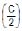+ c cos2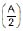=3b/2, then the sides a, b and c

Solution:
QUESTION: 10

In a triangle ABC, medians AD and BE are drawn. If AD = 4, ∠DAB = π/6 and ∠ABE =π/3, then the area of the ΔABC is

Solution: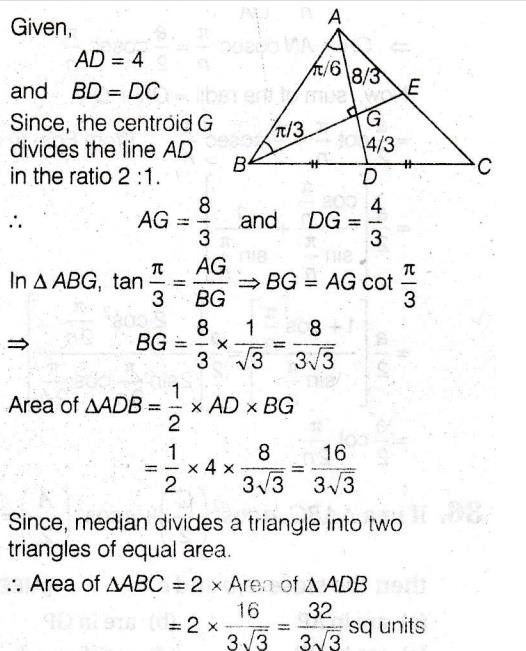QUESTION: 11

If the radius of the circumcircle of an isosceles triangle PQR is equal to PQ = PR then the angle P is

Solution:
QUESTION: 12

Given an isosceles triangle, whose one angle is 120º and radius of its incircle is √3, then the area of the triangle in sq. units is

Solution:

Let the angles be A,B,C

Given:∠A=120∘

and since it is an isosceles Triangle, other two angles must be equal

We have B=C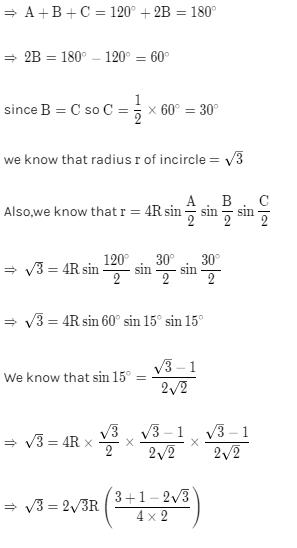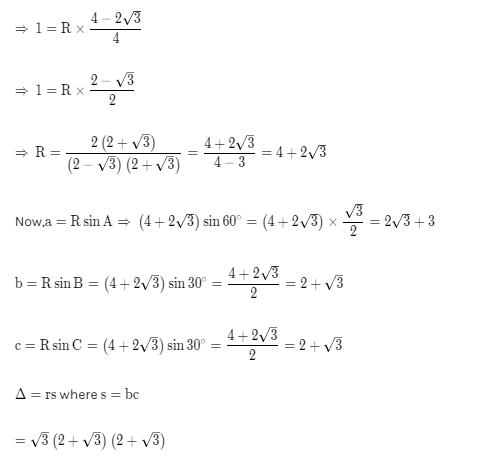= √3(4 + 3 + 4√3)

= 7√3 + 12

QUESTION: 13

The sides a, b, c of a triangle ABC are the roots of x3 – 11x2 + 38x – 40 = 0, then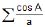=

Solution:
QUESTION: 14

In a triangle ABC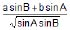= 4, ∠C = π/3, then a2 + b2 – c2 =

Solution:
QUESTION: 15

In a triangle cot A : cot B : cot C = 30 : 19 : 6, then a : b : c

Solution:
QUESTION: 16

If twice the square of the diameter of a circle is equal to sum of the squares of the sides of the inscribed triangle ABC, then sin2A + sin2B + sin2C is equal to

Solution:
QUESTION: 17

In a triangle ABC if  a/1 = b/√3 = c/2, then

Solution:
QUESTION: 18

In a triangle ABC, if s – a, s – b, s – c are in GP, then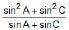=

Solution:
QUESTION: 19

If cos A =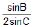, then ΔABC is

Solution:
QUESTION: 20

In a triangle ABC if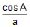=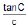, then sin (B+C) is equal to

Solution:
QUESTION: 21

In a triangle ABC, 1 – tan (A/2) tan (B/2) is equal to

Solution:
QUESTION: 22

The angles of a triangle ABC are in A.P. The largest angle is twice the smallest angle and the median to the largest side divides the angle at the vertex in the ratio 2 : 3. If length of the median in 2√3 cm, length of the largest side is

Solution:
QUESTION: 23

The vertices angle of a triangle is divided into two parts, such that the tangent of one part is 3 times the tangent of the other and the difference of these parts is 30º, then the triangle is

Solution:
QUESTION: 24

In a triangle ABC, if tan (A/2) = p, tan (B/2) = q, then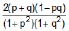is equal to

Solution:
QUESTION: 25

If I is the incentre of a triangle whose in raidus and circumradius are r and R respectively; I1 I2 I3 is its ex-centre triangle, then I I1 . I I2 . I I3 is equal to

Solution:
QUESTION: 26

If R is the circumradius of a triangle ABC then the area of its pedal triangle is

Solution:
QUESTION: 27

In an isosceles triangle with base angle a and lateral side 4, Rr =

Solution: